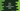# 5 ways in Dart to print the multiplication table## Write a dart program to print the multiplication table for a given number:

This post will show you how to write a program in dart to print the multiplication table for any given number. We can take the number as an input from the user or we can use a constant number.

We need to use a loop to write this program. You will learn how to take user inputs and how to use different loops in dart.

### Method 1: By using a for loop:

We can use a for loop to print the table. The syntax of for loop is:

``````for(<initialization>, <condition>, <end-operation>){
//loop content
}``````

It has three parts.

• In the initialization, we initialize a constant.
• In the condition, it checks for a condition and keeps running the content of the loop until this condition is true.
• In the end-operation, it performs certain operations at the end of each iteration.

We can use a for loop to print the multiplication table for a number as below:

``````void main() {
int n = 10;

for (var i = 1; i <= 10; i++) {
print("\${n}*\${i}=\${n * i}");
}
}``````

If you run this program, it will print:

``````10*1=10
10*2=20
10*3=30
10*4=40
10*5=50
10*6=60
10*7=70
10*8=80
10*9=90
10*10=100``````

This program can be changed to take the number as input from the user:

``````import 'dart:io';

void main() {
print("Enter a number: ");

for (var i = 1; i <= 10; i++) {
print("\${n}*\${i}=\${n * i}");
}
}``````

It will print:

``````Enter a number:
12
12*1=12
12*2=24
12*3=36
12*4=48
12*5=60
12*6=72
12*7=84
12*8=96
12*9=108
12*10=120``````

### Method 2: By using a while loop:

Similar to for-loops, we can also use while loops in dart. The while loop checks for a condition and runs until the condition is true. The following program uses a while loop to print the multiplication table for a number:

``````import 'dart:io';

void main() {
print("Enter a number: ");

var i = 1;
while (i <= 10) {
print("\${n}*\${i}=\${n * i}");
i++;
}
}``````

You can see that we are initializing the value of i before the loop starts and its value is incremented by 1 at the end of each iteration. The loop runs until the value of i is less than or equal to 10.

It will print similar output as the previous example.

``````Enter a number:
13
13*1=13
13*2=26
13*3=39
13*4=52
13*5=65
13*6=78
13*7=91
13*8=104
13*9=117
13*10=130``````

### By using do..while loop:

The do..while loop runs the code in the do block and then it checks the while condition. The following program uses do..while loop to print the multiplication table:

``````import 'dart:io';

void main() {
print("Enter a number: ");

var i = 1;
do {
print("\${n}*\${i}=\${n * i}");
i++;
} while (i <= 10);
}``````

Output:

``````Enter a number:
9
9*1=9
9*2=18
9*3=27
9*4=36
9*5=45
9*6=54
9*7=63
9*8=72
9*9=81
9*10=90``````

### Method 4: By using for..in loop:

The for..in loop takes one iterator and iterates over the items of the iterator. We can create one list of numbers from 1 to 10 and iterate over the list to print the multiplication table.

``````import 'dart:io';

void main() {
print("Enter a number: ");

var numberList = List<int>.generate(10, (i) => i + 1);

for (var i in numberList) {
print("\${n}*\${i}=\${n * i}");
}
}``````

The numberList is the list of integers 1, 2, 3, 4, 5, 6, 7, 8, 9, 10. The for..in loop iterates over this list and prints the multiplication table for the given number.

It will print:

``````Enter a number:
6
6*1=6
6*2=12
6*3=18
6*4=24
6*5=30
6*6=36
6*7=42
6*8=48
6*9=54
6*10=60``````

### Method 5: By using forEach loop:

Similar to the for..in loop, we can also use the forEach loop. It will iterate over the list elements to print the multiplication table:

``````import 'dart:io';

void main() {
print("Enter a number: ");

var numberList = List<int>.generate(10, (i) => i + 1);

numberList.forEach((var i) => print("\${n}*\${i}=\${n * i}"));
}``````

Output:

``````Enter a number:
3
3*1=3
3*2=6
3*3=9
3*4=12
3*5=15
3*6=18
3*7=21
3*8=24
3*9=27
3*10=30``````